Share

# HC Verma solutions for Class 12 Concepts of Physics 1 chapter 9 - Centre of Mass, Linear Momentum, Collision [Latest edition]

Textbook page

#### Chapters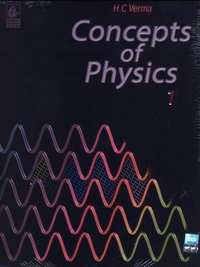## Chapter 9: Centre of Mass, Linear Momentum, Collision

#### HC Verma solutions for Class 12 Concepts of Physics 1 Chapter 9 Centre of Mass, Linear Momentum, Collision Exercise Short Answers [Pages 156 - 157]

Short Answers | Q 1 | Page 156

Can the centre of mass of a body be at a point outside the body?

Short Answers | Q 2 | Page 156

If all the particles of a system lie in X-Y plane, is it necessary that the centre of mass be in X-Y plane?

Short Answers | Q 3 | Page 156

If all the particle of a system lie in a cube, is it necessary that the centre of mass be in the cube?

Short Answers | Q 4 | Page 156

The centre of mass is defined as $\vec{R} = \frac{1}{M} \sum_i m_i \vec{r_i}$. Suppose we define "centre of charge" as $\vec{R}_c = \frac{1}{Q} \sum_i q_i \vec{r_i}$ where qi represents the ith charge placed at $\vec{r}_i$ and Q is the total charge of the system.
(a) Can the centre of charge of a two-charge system be outside the line segment joining the charges?
(b) If all the charges of a system are in X-Y plane, is it necessary that the centre of charge be in X-Y plane?
(c) If all the charges of a system lie in a cube, is it necessary that the centre of charge be in the cube?

Short Answers | Q 5 | Page 156

The weight Mg of an extended body is generally shown in a diagram to act through the centre of mass. Does it mean that the earth does not attract other particles?

Short Answers | Q 6 | Page 156

A bob suspended from the ceiling of a car which is accelerating on a horizontal road. The bob stays at rest with respect to the car with the string making an angle θ with the vertical. The linear momentum of the bob as seen from the road is increasing with time. Is it a violation of conservation of linear momentum? If not, where is the external force changes the linear momentum?

Short Answers | Q 7 | Page 156

You are waiting for a train on a railway platform. Your three-year-old niece is standing on your iron trunk containing the luggage. Why does the trunk not recoil as she jumps off on the platform?

Short Answers | Q 8 | Page 156

In a head-on collision between two particles, is it necessary that the particles will acquire a common velocity at least for one instant?

Short Answers | Q 9 | Page 156

A collision experiment is done on a horizontal table kept in an elevator. Do you expect a change in the result if the elevator is accelerated up or down because of the noninertial character of the frame?

Short Answers | Q 10 | Page 156

Two bodies make an elastic head-on collision on a smooth horizontal table kept in a car. Do you expect a change in the result if the car is accelerated in a horizontal road because of the non inertial character of the frame? Does the equation "Velocity of separation = Velocity of approach" remain valid in an accelerating car? Does the equation "final momentum = initial momentum" remain valid in the accelerating car?

Short Answers | Q 11 | Page 156

If the total mechanical energy of a particle is zero, is its linear momentum necessarily zero? Is it necessarily nonzero?

Short Answers | Q 12 | Page 156

If the linear momentum of a particle is known, can you find its kinetic energy? If the kinetic energy of a particle is know can you find its linear momentum?

Short Answers | Q 13 | Page 156

What can be said about the centre of mass of a uniform hemisphere without making any calculation? Will its distance from the centre be more than r/2 or less than r/2?

Short Answers | Q 14 | Page 156

You are holding a cage containing a bird. Do you have to make less effort if the bird flies from its position in the cage and manages to stay in the middle without touching the walls of the cage? Does it makes a difference whether the cage is completely closed or it has rods to let air pass?

Short Answers | Q 15 | Page 157

A fat person is standing on a light plank floating on a calm lake. The person walks from one end to the other on the plank. His friend sitting on the shore watches him and finds that the person hardly moves any distance because the plank moves backward about the same distance as the person moves on the plank. Explain.

Short Answers | Q 16 | Page 156

A high-jumper successfully clears the bar. Is it possible that his centre of mass crossed the bar from below it? Try it with appropriate figures.

Short Answers | Q 17 | Page 157

Which of the two persons shown in figure is more likely to fall down? Which external force is responsible for his falling down?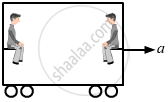Short Answers | Q 18 | Page 157

Suppose we define a quantity 'Linear momentum' as linear momentum = mass × speed.
The linear momentum of a system of particles is the sum of linear momenta of the individual particles. Can we state principle of conservation of linear momentum as "linear momentum of a system remains constant if no external force acts on it"?

Short Answers | Q 19 | Page 157

Use the definition of linear momentum from the previous question. Can we state the principle of conservation of linear momentum for a single particle?

Short Answers | Q 20 | Page 157

To accelerate a car we ignite petrol in the engine of the car. Since only an external force can accelerate the centre of mass, is it proper to say that "the force generated by the engine accelerates the car"?

Short Answers | Q 21 | Page 157

A ball is moved on a horizontal table with some velocity. The ball stops after moving some distance. Which external force is responsible for the change in the momentum of the ball?

Short Answers | Q 22 | Page 157

Consider the situation of the previous problem. Take "the table plus the ball" as the system. friction between the table and the ball is then an internal force. As the ball slows down, the momentum of the system decreases. Which external force is responsible for this change in the momentum?

Short Answers | Q 23 | Page 157

When a nucleus at rest emits a beta particle, it is found that the velocities of the recoiling nucleus and the beta particle are not along the same straight line. How can this be possible in view of the principle of conservation of momentum?

Short Answers | Q 24 | Page 157

A van is standing on a frictionless portion of a horizontal road. To start the engine, the vehicle must be set in motion in the forward direction. How can be persons sitting inside the van do it without coming out and pushing from behind?

Short Answers | Q 25 | Page 157

In one-dimensional elastic collision of equal masses, the velocities are interchanged. Can velocities in a one-dimensional collision be interchanged if the masses are not equal?

#### HC Verma solutions for Class 12 Concepts of Physics 1 Chapter 9 Centre of Mass, Linear Momentum, Collision Exercise MCQ [Pages 157 - 158]

MCQ | Q 1 | Page 157

Consider the following the equations
(A) $\vec{R} = \frac{1}{M} \sum_i m_i \vec{r_i}$ and
(B) $\vec{a}_{CM} = \frac{\vec{F}}{M}$
In a noninertial frame

• both are correct

• both are wrong

•  A is correct but B is wrong

•  B is correct but A is wrong.

MCQ | Q 2 | Page 157

Consider the following two statements:

(A) Linear momentum of the system remains constant.

(B) Centre of mass of the system remains at rest.

• A implies B and B implies A.

• A does not imply B and B does not imply A.

• A implies B but B does not imply A.

• B implies A but A does not imply B.

MCQ | Q 3 | Page 157

Consider the following two statements:

(A) Linear momentum of a system of particles is zero.

(B) Kinetic energy of a system of particles is zero.

• A implies B and B implies A.

• A does not imply B and B does not imply A.

• A implies B but B does not imply A.

• B implies A but A does not imply B.

MCQ | Q 4 | Page 157

Consider the following two statements:

(A)  The linear momentum of a particle is independent of the frame of reference.

(B) The kinetic energy of a particle is independent of the frame of reference.

•  Both A and B are true.

• A is true but B is false.

• A is false but B is true.

• both A and B are false.

MCQ | Q 5 | Page 157

All the particles of a body are situated at a distance R from the origin. The distance of the centre of mass of the body from the origin is

• = R

•  ≤ R

•  > R

• ≥ R

MCQ | Q 6 | Page 157

A circular plate of diameter d is kept in contact with a square plate of edge d as show in figure. The density of the material and the thickness are same everywhere. The centre of mass of the composite system will be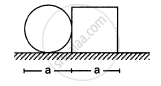• inside the circular plate

• inside the square plate

• at the point of contact

• outside the system.

MCQ | Q 7 | Page 158

Consider a system of two identical particles. One of the particles is at rest and the other has an acceleration a. The centre of mass has an Acceleration

• zero

• $\frac{1}{2} \vec{a}$

• $\vec{a}$

• $2 \vec{a}$

MCQ | Q 8 | Page 158

Internal forces can change

• the linear momentum but not the kinetic energy

•  the kinetic energy but not the linear momentum

•  linear momentum as well as kinetic energy

•  neither the linear momentum nor the kinetic energy

MCQ | Q 9 | Page 158

A bullet hits a block kept at rest on a smooth horizontal surface and gets embedded into it. Which of the following does not change?

• linear momentum of the block

• kinetic energy of the block

• gravitational potential energy of the block

•  temperature of the block.

Q 10 | Page 158

A uniform sphere is placed on a smooth horizontal surface and a horizontal force F is applied on it at a distance h above the surface. The acceleration of the centre

• is maximum when h = 0

• is maximum when h = R

•  is maximum when h = 2R

•  is independent of h.

MCQ | Q 11 | Page 158

A body falling vertically downwards under gravity breaks in two parts of unequal masses. The centre of mass of the two parts taken together shifts horizontally towards

• heavier piece

•  lighter piece

•  does not shift horizontally

• depends on the vertical velocity at the time of breaking.

MCQ | Q 12 | Page 158

A ball kept in a closed box moves in the box making collisions with the walls. The box is kept on a smooth surface. The velocity of the centre of mass

• of the box remains constant

• of the box plus the ball system remains constant

• of the ball remains constant

•  of the ball relative to the box remains constant.

MCQ | Q 13 | Page 158

A body at rest breaks into two pieces of equal masses. The parts will move

• in same direction

•  along different lines

• in opposite directions with equal speeds

•  in opposite directions with unequal speeds.

MCQ | Q 14 | Page 158

A heavy ring of mass m is clamped on the periphery of al light circular disc. A small particle having equal mass is clamped at the centre of the disc. The system is rotated in such a way that the centre moves in a circle of radius r with a uniform speed v. We conclude that an external force

• $\frac{m v^2}{r}$ must be acting on the central particle

• $\frac{2m v^2}{r}$ must be acting on the central particle

• $\frac{2m v^2}{r}$ must be acting on the system

• $\frac{2m v^2}{r}$ must be acting on the ring.

MCQ | Q 15 | Page 158

The quantities remaining constant in a collisions are

• momentum, kinetic energy and temperature

•  momentum and kinetic energy but not temperature

• momentum and temperature but not kinetic energy

• momentum, but neither kinetic energy nor temperature.

MCQ | Q 16 | Page 158

A nucleus moving with a velocity $\vec{v}$ emits an α-particle. Let the velocities of the α-particle and the remaining nucleus be v1 and v2 and their masses be m1 and m2

• $\vec{v} , \vec{v}_1 \text{ and } \vec{v}_2$ must be parallel to each other.

• None of the two of $\vec{v} , \vec{v}_1 \text{ and } \vec{v}_2$ should be parallel to each other.

• $\vec{v_1} + \vec{v_2}$ must be parallel to $\vec{v}$

• $m_1 \vec{v_1} + m_2 \vec{v_2}$  must be parallel to $\vec{v}$

MCQ | Q 17 | Page 158

A shell is fired from a cannon with a velocity V at an angle θ with the horizontal direction. At the highest point in its path, it explodes into two pieces of equal masses. One of the pieces retraces its path to the cannon. The speed of the other piece immediately after the explosion is

•  3V cos θ

• 2V cos θ

• $\frac{3}{2}$  V cos θ

• V cos θ

MCQ | Q 18 | Page 158

In an elastic collision

•  the initial kinetic energy is equal to the final kinetic energy

•  the final kinetic energy is less than the initial kinetic energy

• the kinetic energy remains constant

•  the kinetic energy first increases then decreases.

MCQ | Q 19 | Page 158

In an elastic collision

• the initial kinetic energy is equal to the final kinetic energy

• the final kinetic energy is less than the initial kinetic energy

• the kinetic energy remains constant

• the kinetic energy first increases then decreases.

#### HC Verma solutions for Class 12 Concepts of Physics 1 Chapter 9 Centre of Mass, Linear Momentum, Collision Exercise MCQ [Pages 158 - 159]

MCQ | Q 1 | Page 158

The centre of mass of a system of particles is at the origin. It follows that

•  the number of particles to the right of the origin is equal to the number of particles to the left

•  the total mass of the particles to the right of the origin is same as the total mass to the left of the origin

• the number of particles on X-axis should be equal to the number of particles on Y-axis

•  if there is a particle on the positive X-axis, there must be at least one particle on the negative X-axis

• none of these

MCQ | Q 2 | Page 159

A body has its centre of mass at the origin. the x-coordinates of the particles

(a) may be all positive
(b) may be all negative
(c) may be all non-negative
(d) may be positive for some cases and negative in other cases.

MCQ | Q 3 | Page 158

In which of the following cases the centre of mass of a rod is certainly not at its centre?
(a) the density continuously increases from left to right
(b) the density continuously decreases from left to right
(c) the density decreases from left to right upto the centre and then increases
(d) the density increases from left to right upto the centre and then decreases.

MCQ | Q 4 | Page 159

If the external force acting on a system have zero resultant, the centre of mass
(a) must not move
(b) must not accelerate
(c) may move
(d) may accelerate.

MCQ | Q 5 | Page 159

A nonzero external force acts on a system of particles. The velocity and the acceleration of the centre of mass are found to be v0 and a0 at instant t. It is possible that
(a) v0 = 0, a0 = 0
(b) v0 = 0, a0 ≠ 0
(c) v0 ≠ 0, a0 = 0
(d) v0 ≠ 0, a0 ≠ 0

MCQ | Q 6 | Page 159

Two balls are thrown simultaneously in air. The acceleration of the centre of mass of the two balls while in air

• depends on the direction of the motion of the balls

• depends on the masses of the two balls

• depends on the speeds of the two balls

• is equal to g.

MCQ | Q 7 | Page 159

A block moving in air breaks in two parts and the parts separate
(a) the total momentum must be conserved
(b) the total kinetic energy must be conserved
(c) the total momentum must change
(d) the total kinetic energy must change

MCQ | Q 8 | Page 159

In an elastic collision
(a) the kinetic energy remains constant
(b) the linear momentum remains constant
(c) the final kinetic energy is equal to the initial kinetic energy
(d) the final linear momentum is equal to the initial linear momentum.

MCQ | Q 9 | Page 159

A ball hits a floor and rebounds after an inelastic collision. In this case
(a) the momentum of the ball just after the collision is same as that just before the collision
(b) the mechanical energy of the ball remains the same during the collision
(c) the total momentum of the ball and the earth is conserved
(d) the total energy of the ball and the earth remains the same

MCQ | Q 10 | Page 159

A body moving towards a finite body at rest collides with it. It is possible that
(a) both the bodies come to rest
(b) both the bodies move after collision
(c) the moving body comes to rest and the stationary body starts moving
(d) the stationary body remains stationary, the moving body changes its velocity.

MCQ | Q 11 | Page 159

In a head-on elastic collision of two bodies of equal masses
(a) the velocities are interchanged
(b) the speeds are interchanged
(c) the momenta are interchanged
(d) the faster body slows down and the slower body speeds up.

#### HC Verma solutions for Class 12 Concepts of Physics 1 Chapter 9 Centre of Mass, Linear Momentum, Collision [Pages 159 - 164]

Q 1 | Page 159

Three particles of masses 1.0 kg, 2.0 kg and 3.0 kg are placed at the corners A, B and C respectively of an equilateral triangle ABC of edge 1 m. Locate the centre of mass of the system.

Q 2 | Page 159

The structure of a water molecule is shown in figure. Find the distance of the centre of mass of the molecule from the centre of the oxygen atom.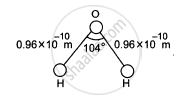Q 3 | Page 159

Seven homogeneous bricks, each of length L, are arranged as shown in figure. Each brick is displaced with respect to the one in contact by L/10. Find the x-coordinate fo the centre of mass relative to the origin shown.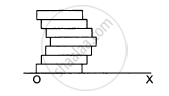Q 4 | Page 159

A uniform disc of radius R is put over another uniform disc of radius 2R of the same thickness and density. The peripheries of the two discs touch each other. Locate the centre of mass of the system.

Q 5 | Page 160

A disc of radius R is cut out from a larger disc of radius 2R in such a way that the edge of the hole touches the edge of the disc. Locate the centre of mass of the residual disc.

Q 6 | Page 160

A square plate of edge d and a circular disc of diameter d are placed touching each other at the midpoint of an edge of the plate as shown in figure. Locate the centre of mass of the combination, assuming same mass per unit area for the two plates.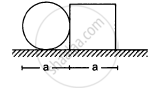Q 7 | Page 160

Calculate the velocity of the centre of mass of the system of particles shown in figure.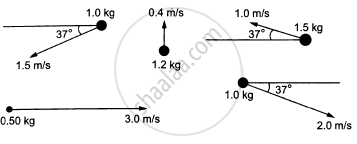Q 8 | Page 160

Two blocks of masses 10 kg and 20 kg are placed on the X-axis. The first mass is moved on the axis by a distance of 2 cm. By what distance should the second mass be moved to keep the position of the centre of mass  unchanged?

Q 9 | Page 160

Two blocks of masses 10 kg and 30 kg are placed along a vertical line. The first block is raised through a height of 7 cm. By what distance should the second mass be moved to raise the centre of mass by 1 cm?

Q 10 | Page 160

Consider a gravity-free hall in which a tray of mass M, carrying a cubical block of ice of mass m and edge L, is at rest in the middle. If the ice melts, by what distance does the centre of mass of "the tray plus the ice" system descend?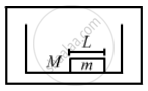Q 11 | Page 160

Find the centre of mass of a uniform plate having semicircular inner and outer boundaries of radii R1 and R2.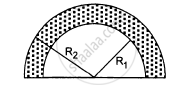Q 12 | Page 160

Mr. Verma (50 kg) and Mr. Mathur (60 kg) are sitting at the two extremes of a 4 m long boat (40 kg) standing still in water. To discuss a mechanics problem, they come to the middle of the boat. Neglecting friction with water, how far does the boat move on the water during the process?

Q 13 | Page 160

A car of mass M is at rest on a frictionless  horizontal surface and a pendulum bob of mass m hangs from the roof of the cart. The string breaks, the bob falls on the floor, makes serval collisions on the floor and finally lands up in a small slot made in the floor. The horizontal distance between the string and the slot is L. Find the displacement of the cart during this process.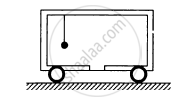Q 14 | Page 160

The balloon, the light rope and the monkey shown in figure are at rest in the air. If the monkey reaches the top of the rope, by what distance does the balloon descend? Mass of the balloon = M, mass of the monkey = m and the length of the rope ascended by the monkey = L.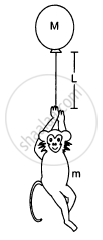Q 15 | Page 160

Find the ratio of the linear momenta of two particles of masses 1.0 kg and 4.0 kg if their kinetic energies are equal.

Q 16 | Page 160

A uranium-238 nucleus, initially at rest, emits an alpha particle with a speed of 1.4 × 107m/s. Calculate the recoil speed of the residual nucleus thorium-234. Assume that the mass of a nucleus is proportional to the mass number.

Q 17 | Page 160

A man of mass 50 kg starts moving on the earth and acquires a speed 1.8 m/s. With what speed does the earth recoil? Mass of earth = 6 × 1024 kg.

Q 18 | Page 160

A neutron initially at rest, decays into a proton, an electron and an antineutrino. The ejected electron has a momentum of 1.4 × 10−26 kg-m/s and the antineutrino 6.4 × 10−27kg-m/s. Find the recoil speed of the proton (a) if the electron and the antineutrino are ejected along the same direction and (b) if they are ejected along perpendicular directions. Mass of the proton = 1.67 × 10−27 kg.

Q 19 | Page 161

A man of mass M having a bag of mass m slips from the roof of a tall building of height H and starts falling vertically in the following figure. When at a height h from the ground, the notices that the ground below him is pretty hard, but there is a pond at a horizontal  distance x from the line of fall. In order to save himself he throws the bag horizontally (with respect to himself) in the direction opposite to the pond. Calculate the minimum horizontal velocity imparted to the bag so that the man lands in the water. If the man just succeeds to avoid the hard ground, where will the bag land?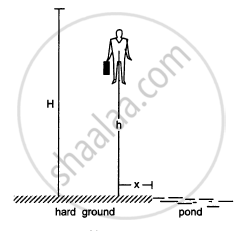Q 20 | Page 161

A ball of mass 50 g moving at a speed of 2.0 m/s strikes a plane surface at an angle of incidence 45°. The ball is reflected by the plane at equal angle of reflection with the same speed. Calculate (a) the magnitude of the change in momentum of the ball (b) the change in the magnitude of the momentum of the ball.

Q 21 | Page 161

Light in certain cases may be considered as a stream of particles called photons. Each photon has a linear momentum h/λ where h is the Planck's constant and λ is the wavelength of the light. A beam of light of wavelength λ is incident on a plane mirror at an angle of incidence θ. Calculate the change in the linear momentum of a photon as the beam is reflected by the mirror.

Q 22 | Page 161

A block at rest explodes into three equal parts. Two parts start moving along X and Y axes respectively with equal speeds of 10 m/s. Find the initial velocity of the third part.

Q 23 | Page 161

Two fat astronauts each of mass 120 kg are travelling in a closed  spaceship moving at a speed of 15 km/s in the outer space far removed from all other material objects. The total mass of the spaceship and its contents including the astronauts is 660 kg. If the astronauts do slimming exercise and thereby reduce their masses to 90 kg each, with what velocity will the spaceship move?

Q 24 | Page 161

During a heavy rain, hailstones of average size 1.0 cm in diameter fall with an average speed of 20 m/s. Suppose 2000 hailstones strike every square meter of a 10 m × 10 m roof perpendicularly in one second and assume that the hailstones do not rebound. Calculate the average force exerted by the falling hailstones on the roof. Density of a hailstone is 900 kg/m3.

Q 25 | Page 161

A ball of mass m is dropped onto a floor from a certain height. The collision is perfectly elastic and the ball rebounds to the same height and again falls. Find the average force exerted by the ball on the floor during a long time interval.

Q 26 | Page 161

A railroad car of mass M is at rest on frictionless rails when a man of mass m starts moving on the car towards the engine. If the car recoils with a speed v backward on the rails, with what velocity is the man approaching the engine?

Q 27 | Page 161

A gun is mounted on a railroad car. The mass of the car, the gun, the shells and the operator is  50 m where m is the mass of one shell. If the velocity of the shell with respect to the gun (in its state before firing) is 200 m/s, what is the recoil speed of the car after the second shot? Neglect friction.

Q 28 | Page 161

Two persons each of mass m are standing at the two extremes of a railroad car of mass M resting on a smooth track(In the following figure). The person on left jumps to the left with a horizontal speed u with respect to the state of the car before the jump. Thereafter, the other person jumps to the right, again with the same horizontal speed u with respect to the state of the car before his jump. Find the velocity of the car after both the persons have jumped off.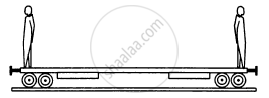Q 29 | Page 161

Figure shows a small block of mass m which is started with a speed v on the horizontal part of the bigger block of mass M placed on a horizontal floor. The curved part of the surface shown in semicircular. All the surfaces are frictionless. Find the speed of the bigger block when the smaller block reaches the point A of the surface.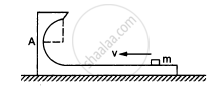Q 30 | Page 162

In a typical Indian Bugghi (a luxury cart drawn by horses), a wooden plate is fixed on the rear on which one person can sit. A bugghi of mass 200 kg is moving at a speed of 10 km/h. As it overtakes a school boy walking at a speed of 4 km/h, the boy sits on the wooden plate. If the mass of the boy is 25 kg, what will be the plate. If the mass of the boy is 25 kg, what will be the new velocity of the bugghi ?

Q 31 | Page 162

A ball of mass 0.50 kg moving at a speed of 5.0 m/s collides with another ball of mass 1.0 kg. After the collision the balls stick together and remain  motionless. What was the velocity of the 1.0 kg block before the collision?

Q 32 | Page 162

A 60 kg man skating with a speed of 10 m/s collides with a 40 kg skater at rest and they cling to each other. Find the loss of kinetic energy during the collision.

Q 33 | Page 162

Consider a head-on collision between two particles of masses m1 and m2. The initial speeds of the particles are u1 and u2 in the same direction. the collision starts at t = 0 and the particles interact for a time interval ∆t. During the collision, the speed of the first particle varies as $v(t) = u_1 + \frac{t}{∆ t}( v_1 - u_1 )$
Find the speed of the second particle as a function of time during the collision.

Q 34 | Page 162

A bullet of mass m moving at a speed v hits a ball of mass M kept at rest. A small part having mass m breaks from the ball and sticks to the bullet. The remaining ball is found to move at a speed v1 in the direction of the bullet. Find the velocity of the bullet after the collision.

Q 35 | Page 162

A ball of mass m moving at a speed v makes a head-on collision with an identical ball at rest. The kinetic energy of the balls after the collision is three fourths of the original. Find the coefficient of restitution.

Q 36 | Page 162

A block of mass 2.0 kg moving 2.0 m/s collides head on with another block of equal mass kept at rest. (a) Find the maximum possible loss in kinetic energy due to the collision. (b) If he actual loss in kinetic energy is half of this maximum, find the coefficient of restitution.

Q 37 | Page 162

A particle of mass 100 g moving at an initial speed u collides with another particle of same mass kept initially at rest. If the total kinetic energy becomes 0.2 J after the collision, what could be the minimum and the maximum value of u.

Q 38 | Page 162

Two friends A and B (each weighing 40 kg) are sitting on a frictionless platform some distance d apart. A rolls a ball of mass 4 kg on the platform towards B which B catches. Then B rolls the ball towards A and A catches it. The ball keeps on moving back and forth between A and B. The ball has a fixed speed of 5 m/s on the platform. (a) Find the speed of A after he catches the ball for the first time. (c) Find the speeds of A and Bafter the all has made 5 round trips and is held by A. (d) How many times can A roll the ball? (e) Where is the centre of mass of the system "A + B + ball" at the end of the nth trip?

Q 39 | Page 162

A ball falls on the ground from a height of 2.0 m and rebounds up to a height of 1.5 m. Find the coefficient of restitution.

Q 40 | Page 162

In a gamma decay process, the internal energy of a nucleus of mass M decreases, a gamma photon of energy E and linear momentum E/c is emitted and the nucleus recoils. Find the decrease in internal energy.

Q 41 | Page 162

A block of mass 2.0 kg is moving on a frictionless horizontal surface with a velocity of 1.0 m/s (In the following figure) towards another block of equal mass kept at rest. The spring constant of the spring fixed at one end is 100 N/m. Find the maximum compression of the spring.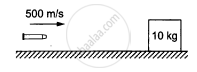Q 42 | Page 162

A bullet of mass 20 g travelling horizontally with a speed of 500 m/s passes through a wooden block of mass 10.0 kg initially at rest on a level surface. The bullet emerges with a speed of 100 m/s and the block slides 20 cm on the surface before coming to rest. Find the friction coefficient between the block and the  surface (See figure).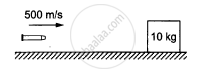Q 43 | Page 162

A projectile is fired with a speed u at an angle θ above a horizontal field. The coefficient of restitution of collision between the projectile and the field is e. How far from the starting point, does the projectile makes its second collision with the field?

Q 44 | Page 162

A ball falls on an inclined plane of inclination θ from a height h above the point of impact and makes a perfectly elastic collision. Where will it hit the plane again?

Q 45 | Page 162

Solve the previous problem if the coefficient of restitution is e. $\text{ Use } \theta = 45^\circ\, e = \frac{3}{4} \text{ and h = 5 m } .$

Q 46 | Page 162

A block of mass 200 g is suspended through a vertical spring. The spring is stretched by 1.0 cm when the block is in equilibrium. A particle of mass 120 g is dropped on the block from a height of 45 cm. The particle sticks to the block after the impact. Find the maximum extension of the spring. Take g = 10 m/s2.

Q 47 | Page 163

A bullet of mass 25 g is fired horizontally into a ballistic pendulum of mass 5.0 kg and gets embedded in it. If the centre of the pendulum rises by a distance of 10 cm, find the speed of the bullet.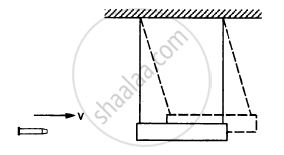Q 48 | Page 163

A bullet of mass 20 g moving horizontally at a speed of 300 m/s is fired into a wooden block of mass 500 g suspended by a long string. The bullet crosses the block and emerges on the other side. If the centre of mass of the block rises through a height of 20.0 cm, find the speed of the bullet as it emerges from the block.

Q 49 | Page 163

Two mass m1 and m2 are connected by a spring of spring constant k and are placed on a frictionless horizontal surface. Initially the spring is stretched through a distance x0 when the system is released from rest. Find the distance moved by the two masses before they again come to rest.

Q 50 | Page 163

Two blocks of masses m1 and m2 are connected by a spring of spring constant k (See figure). The block of mass m2 is given a sharp impulse so that it acquires a velocity v0 towards right. Find (a) the velocity of the centre of mass, (b) the maximum elongation that the spring will suffer.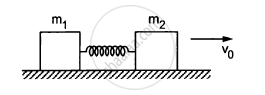Q 51 | Page 163

Consider the situation of the previous problem. Suppose each of the blocks is pulled by a constant force F instead of any impulse. Find the maximum elongation that the spring will suffer and the distance moved by the two blocks in the process.

Q 52 | Page 163

Consider the situation of the previous problem. Suppose the block of mass m1 is pulled by a constant force F1 and the other block is pulled by a constant force F2. Find the maximum elongation that the spring will suffer.

Q 53 | Page 163

Consider a gravity-free hall in which an experimenter of mass 50 kg is resting on a 5 kg pillow, 8 ft above the floor of the hall. He pushes the pillow down so that it starts falling at a speed of 8 ft/s. The pillow makes a perfectly elastic collision with the floor, rebounds and reaches the experimenter's head. Find the time elapsed in the process.

Q 54 | Page 163

The track shown is figure is frictionless. The block B of mass 2m is lying at rest and the block A or mass m is pushed along the track with some speed. The collision between Aand B is perfectly elastic. With what velocity should the block A be started to get the sleeping man awakened?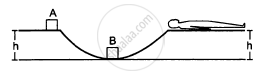Q 55 | Page 163

A bullet of mass 10 g moving horizontally at a speed of 50√7 m/s strikes a block of mass 490 g kept on a frictionless track as shown in figure. The bullet remains inside the block and the system proceeds towards the semicircular track of radius 0.2 m. Where will the block strike the horizontal part after leaving the semicircular track?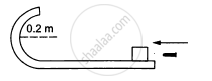Q 56 | Page 163

Two balls having masses m and 2m are fastened to two light strings of same length l (See figure). The other ends of the strings are fixed at O. The strings are kept in the same horizontal line and the system is released from rest. The collision between the balls is elastic. (a) Find the velocity of the balls just after their collision. (b) How high will the ball rise after the collision?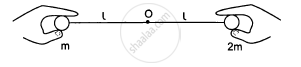Q 57 | Page 163

A uniform chain of mass M and length L is held vertically in such a way that its lower end just touches the horizontal floor. The chain is released from rest in this position. Any portion that strikes the floor comes to test. Assuming that the chain does not form a heap on the floor calculate the force exerted by it on the floor when a length x has reached the floor.

Q 58 | Page 163

The blocks shown in figure have equal masses. The surface of A is smooth but that of Bhas a friction coefficient of 0.10 with the floor. Block A is moving at a speed of 10 m/s towards B which is kept at rest. Find the distance travelled by B if (a) the collision is perfectly elastic and (b) the collision is perfectly inelastic.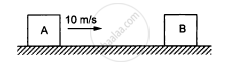Q 59 | Page 163

The friction coefficient between the horizontal surface and each of the block shown in figure is 0.20. The collision between the blocks is perfectly elastic. Find the separation between the two blocks when they come to rest. Take g = 10 m/s2.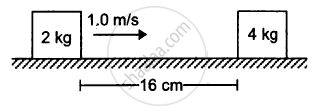Q 60 | Page 163

A block of mass m is placed on a triangular block of mass M which in turn is placed on a horizontal surface as shown in figure. Assuming frictionless surfaces find the velocity of the triangular block when the smaller block reaches the bottom end.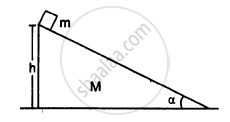Q 61 | Page 163

Figure shows a small body of mass m placed over a larger mass M whose surface is horizontal near the smaller mass and gradually curves to become vertical. The smaller mass is pushed on the longer one at a speed v and the system is left to itself. Assume that all the surface are frictionless. (a) Find the speed of the larger block when the smaller block is sliding on the vertical part. (b) Find the speed of the smaller mass when it breaks off the larger mass at height h. (c) Find the maximum height (from the ground) that the smaller mass ascends. (d) Show that the smaller mass will again land on the bigger one. Find the distance traversed by the bigger block during the time when the smaller block was in its flight under gravity.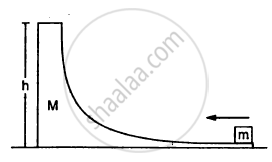Q 62 | Page 164

A small block of superdense material has a mass of 3 ×  1024kg. It is situated at a height h (much smaller than the earth's radius) from where it falls on the earth's surface. Find its speed when its height from the earth's surface has reduce to to h/2. The mass of the earth is 6 × 1024kg.

Q 63 | Page 164

A body of mass m makes an elastic collision with another identical body at rest. Show that if the collision is not head-on the bodies go at right angle to each other after the collision.

Q 64 | Page 164

A small particle travelling with a velocity v collides elastically with a spherical body of equal mass and of radius r initially kept at rest. The centre of this spherical body is located a distance ρ(< r) away from the direction of motion of the particle (see figure below). Find the final velocities of the two particles.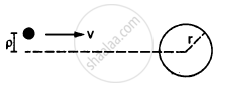[Hint : The force acts along the normal to the sphere
through the contact. Treat the collision as onedimensional
for this direction. In the tangential direction no force acts and the velocities do not change].

## Chapter 9: Centre of Mass, Linear Momentum, Collision## HC Verma solutions for Class 12 Concepts of Physics 1 chapter 9 - Centre of Mass, Linear Momentum, Collision

HC Verma solutions for Class 12 Concepts of Physics 1 chapter 9 (Centre of Mass, Linear Momentum, Collision) include all questions with solution and detail explanation. This will clear students doubts about any question and improve application skills while preparing for board exams. The detailed, step-by-step solutions will help you understand the concepts better and clear your confusions, if any. Shaalaa.com has the CBSE Class 12 Concepts of Physics 1 solutions in a manner that help students grasp basic concepts better and faster.

Further, we at Shaalaa.com provide such solutions so that students can prepare for written exams. HC Verma textbook solutions can be a core help for self-study and acts as a perfect self-help guidance for students.

Concepts covered in Class 12 Concepts of Physics 1 chapter 9 Centre of Mass, Linear Momentum, Collision are Dynamics of Rotational Motion About a Fixed Axis, Angular Momentum in Case of Rotation About a Fixed Axis, Rolling Motion, Momentum Conservation and Centre of Mass Motion, Centre of Mass of a Rigid Body, Centre of Mass of a Uniform Rod, Rigid Body Rotation, Equilibrium of Rigid Bodies, Moment of Inertia, Theorems of Perpendicular and Parallel Axes, Kinematics of Rotational Motion About a Fixed Axis, Motion - Rigid Body, Centre of Mass, Motion of Centre of Mass, Linear Momentum of a System of Particles, Vector Product of Two Vectors, Angular Velocity and Its Relation with Linear Velocity, Torque and Angular Momentum, Equations of Rotational Motion, Comparison of Linear and Rotational Motions, Values of Moments of Inertia for Simple Geometrical Objects (No Derivation).

Using HC Verma Class 12 solutions Centre of Mass, Linear Momentum, Collision exercise by students are an easy way to prepare for the exams, as they involve solutions arranged chapter-wise also page wise. The questions involved in HC Verma Solutions are important questions that can be asked in the final exam. Maximum students of CBSE Class 12 prefer HC Verma Textbook Solutions to score more in exam.

Get the free view of chapter 9 Centre of Mass, Linear Momentum, Collision Class 12 extra questions for Class 12 Concepts of Physics 1 and can use Shaalaa.com to keep it handy for your exam preparation